### Home > CCA2 > Chapter 2 > Lesson 2.1.4 > Problem2-50

2-50.

For each quadratic function below use the method of completing the square or averaging the intercepts to rewrite it in graphing form. Then, state the axis of symmetry and give the vertex of each parabola. Try to use each method at least once.

1. $f(x)=x^2+6x+15$

How many ones do you need to make a perfect square? How many do you have?

$f(x)=(x+3)^2+6$
vertex: $(−3,6)$
axis of symmetry: $x=−3$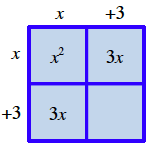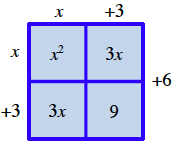2. $y=x^2−4x+9$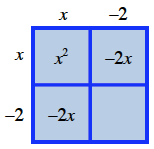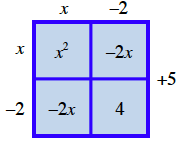3. $f(x)=x^2−8x$

Write each of the terms in its proper location.

How can you write this in the same form as the previous problems?

$- 4 x \quad x ^ { 2 } \quad - 4 \quad x \quad 16 \quad - 24$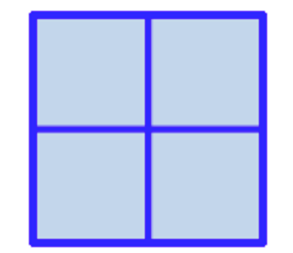4. $y=x^2+7x−2$

$y=(x+3.5)^2−14.25$